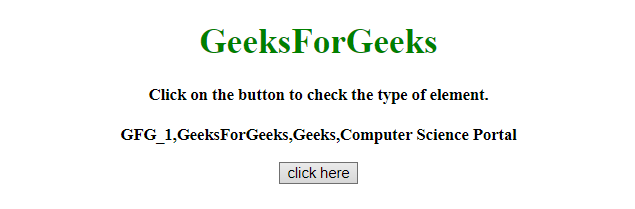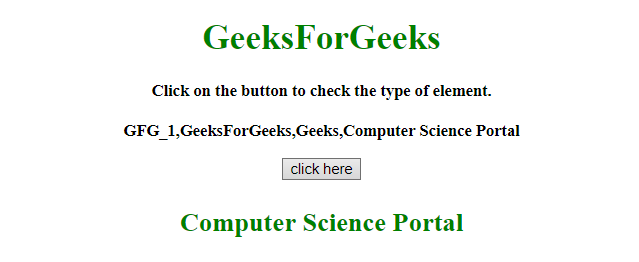# How to select a random element from array in JavaScript ?

• Difficulty Level : Medium
• Last Updated : 14 Jan, 2022

The task is to select the random element from the array using JavaScript.
Approach 1:

• Use Math.random() function to get the random number between(0-1, 1 exclusive).
• Multiply it by the array length to get the numbers between(0-arrayLength).
• Use Math.floor() to get the index ranging from(0 to arrayLength-1).

Example: This example implements the above approach.

## html

 ```<``html``>` `<``head``>``    ``<``title``>``        ``How to select a random element``        ``from array in JavaScript ?``    ````` `<``body` `style = "text-align:center;">``    ` `    ``<``h1` `style = "color:green;">``        ``GeeksForGeeks``    ````    ` `    ``<``p` `id = "GFG_UP" style =``        ``"font-size: 15px; font-weight: bold;">``    ````    ` `    ``<``button` `id = "button" onclick = "GFG_Fun()">``        ``click here``    ````    ` `    ``<``p` `id = "GFG_DOWN" style =``        ``"font-size: 24px; font-weight: bold; color: green;">``    ````    ` `    ``<``script``>``        ``var up = document.getElementById('GFG_UP');``        ``var down = document.getElementById('GFG_DOWN');``        ` `        ``var arr = ["GFG_1", "GeeksForGeeks",``                ``"Geeks", "Computer Science Portal"];``        ` `        ``up.innerHTML = "Click on the button to check "``               ``+ "the type of element.<``br``><``br``>" + arr;``        ` `        ``function GFG_Fun() {``            ``down.innerHTML =``                ``arr[Math.floor(Math.random() * arr.length)];``        ``}``    ````` ``

Output:

• Before clicking on the button:• After clicking on the button:Approach 2:

• The random(a, b) method is used to generates a number between(a to b, b exclusive).
• Taking the floor value to range the numbers from (1 to arrayLength).
• Subtract 1 to get the index ranging from(0 to arrayLength-1).

Example: This example implements the above approach.

## html

 ```<``html``>` `<``head``>``    ``<``title``>``        ``How to select a random element``        ``from array in JavaScript ?``    ````` `<``body` `style = "text-align:center;">``    ` `    ``<``h1` `style = "color:green;">``        ``GeeksForGeeks``    ````    ` `    ``<``p` `id = "GFG_UP" style =``        ``"font-size: 15px; font-weight: bold;">``    ````    ` `    ``<``button` `id = "button" onclick = "GFG_Fun()">``        ``click here``    ````    ` `    ``<``p` `id = "GFG_DOWN" style =``        ``"font-size: 24px; font-weight: bold; color: green;">``    ````    ` `    ``<``script``>``        ``var up = document.getElementById('GFG_UP');``        ``var down = document.getElementById('GFG_DOWN');``        ` `        ``var arr = ["GFG_1", "GeeksForGeeks",``                ``"Geeks", "Computer Science Portal"];``        ` `        ``up.innerHTML = "Click on the button to select"``                        ``+ " random element from the"``                        ``+ " array.<``br``><``br``>" + arr;``        ` `        ``function random(mn, mx) {``            ``return Math.random() * (mx - mn) + mn;``        ``}``        ` `        ``function GFG_Fun() {``            ``down.innerHTML = arr[Math.floor(random(1, 5))-1];``        ``}``    ````` ``

Output:

• Before clicking on the button:• After clicking on the button:JavaScript is best known for web page development but it is also used in a variety of non-browser environments. You can learn JavaScript from the ground up by following this JavaScript Tutorial and JavaScript Examples.

My Personal Notes arrow_drop_up